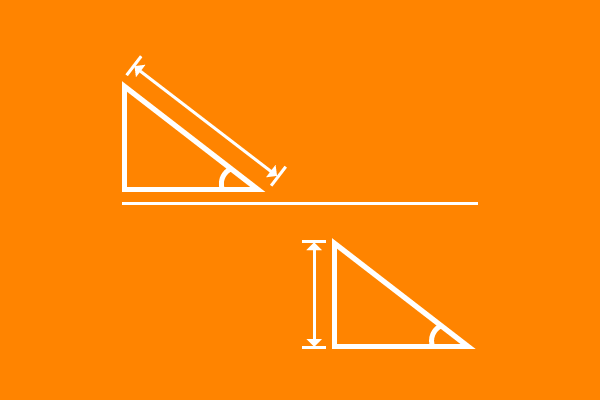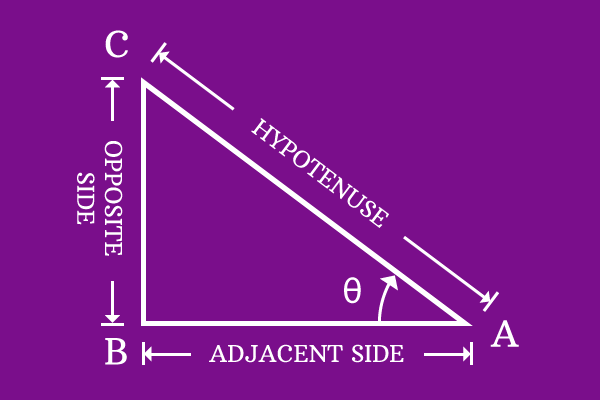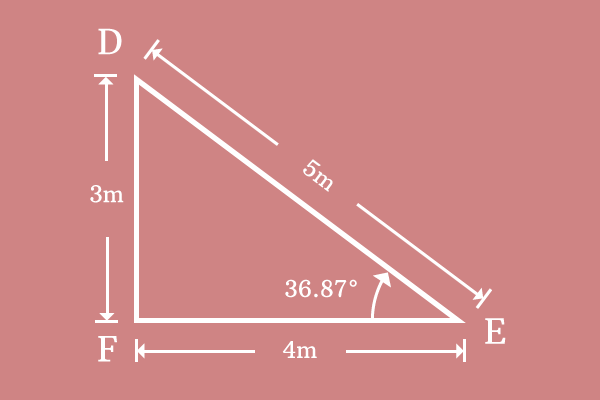# Cosecant

## Definition

A relation of angle with ratio of length of hypotenuse to length of opposite side in a right angled triangle is called cosecant.Cosecant represents the relation of angle of right angled triangle with ratio of length of hypotenuse to length of opposite side of same right angled triangle. It is fundamentally defined from ratio of length of hypotenuse to length of opposite side. Hence, cosecant is called as trigonometric ratio in trigonometry.

The functionality of cosecant represents the relation between angle and ratio of length of hypotenuse to length of opposite side and it is the reason to call it as trigonometric function.

## Mathematical formThe name cosecant was introduced to represent relation of angle with ratio of length of hypotenuse to length of opposite side. The change in angle of right angled triangle also changes the ratio of length of hypotenuse to length of opposite side because they both depend on each other mutually. Hence, cosecant is written as cosecant along with angle to express it in mathematical form but cosecant is written in its short form either $cosec$ or simply $csc$.

In the case of $Δ BAC$, the angle of triangle is $\theta$. So, cosecant along with angle is written as either $cosec \, \theta$ or simply $csc \, \theta$ mathematically. The relation of angle with ratio of length of hypotenuse to length of opposite side in terms of cosecant is written as follows.

$$cosec \, \theta \,\, (or) \,\, csc \, \theta = { Length \, of \, Hypotenuse \over Length \, of \, Opposite \, Side }$$

$\overline{AC}$ and $\overline{BC}$ are hypotenuse and opposite side and lengths of them are $AC$ and $BC$ respectively. Express the equation in terms of lengths of sides of this triangle to express the cosecant in mathematical form.

$$cosec \, \theta \,\, (or) \,\, csc \, \theta = { AC \over BC }$$

### ExampleConsider right angled triangle $DEF$. The lengths of hypotenuse and opposite side and also angle are measured. They are.

1. Length of the hypotenuse $(\overline{DE})$ is $DE = 5$ meters
2. Length of the opposite side $(\overline{DF})$ is $DF = 3$ meters
3. Angle of this right angled triangle is $36.87^°$

$$cosec \, 36.87^° \,\, (or) \,\, csc \, 36.87^° = { DE \over DF }$$

Substitute these three values in the mathematical form expression of co-secant.

$$cosec \, 36.87^° \,\, (or) \,\, csc \, 36.87^° = { 5 \over 3 }$$

$⇒ cosec \, 36.87^° \,\, (or) \,\, csc \, 36.87^° = 1.6667$

The value of ratio of length of hypotenuse to length of opposite side is $1.6667$ at the angle $36.87^°$ in $Δ DEF$. The value $1.6667$ is also known as the value of cosecant at the angle of $36.87^°$ trigonometrically.

Latest Math Topics
Latest Math Problems
Email subscription
Math Doubts is a best place to learn mathematics and from basics to advanced scientific level for students, teachers and researchers. Know more